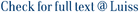In this paper we describe the techniques needed to value securities whose price depends exclusively on the term structure of interest rates. Closed-form formulae are presented for the valuation of bonds and call options on bonds and yields, and the put-call parity used to derive the values of put options. We recall that the value of any financial asset whose price depends exclusively on the term structure of interest rates can be obtained using numerical methods (such as the finite differences method used here). We also describe a method of estimating the parameters of the one-factor CIR model based on non-linear regression techniques (Marquardt’s algorithm). In order to exemplify the method, we applied the model to fixed rate bonds denominated in lire (Italian Treasury bonds - BTPs), ecus (Italian Treasury ecu certificates - CTEs) and dollars (U.S. T-notes and T-bonds) and to Italian Treasury option certificates (CTOs), which are equivalent to combinations of fixed rate bonds and bond options.

The valuation of bonds and bond options: some empirical tests / Barone, Emilio; Cuoco, D.. - (1991), pp. 11-48.

### The valuation of bonds and bond options: some empirical tests

#### Abstract

In this paper we describe the techniques needed to value securities whose price depends exclusively on the term structure of interest rates. Closed-form formulae are presented for the valuation of bonds and call options on bonds and yields, and the put-call parity used to derive the values of put options. We recall that the value of any financial asset whose price depends exclusively on the term structure of interest rates can be obtained using numerical methods (such as the finite differences method used here). We also describe a method of estimating the parameters of the one-factor CIR model based on non-linear regression techniques (Marquardt’s algorithm). In order to exemplify the method, we applied the model to fixed rate bonds denominated in lire (Italian Treasury bonds - BTPs), ecus (Italian Treasury ecu certificates - CTEs) and dollars (U.S. T-notes and T-bonds) and to Italian Treasury option certificates (CTOs), which are equivalent to combinations of fixed rate bonds and bond options.
##### Scheda breve Scheda completa Scheda completa (DC)1991
8820471248
The valuation of bonds and bond options: some empirical tests / Barone, Emilio; Cuoco, D.. - (1991), pp. 11-48.
File in questo prodotto:
Non ci sono file associati a questo prodotto.
##### Pubblicazioni consigliate

I documenti in IRIS sono protetti da copyright e tutti i diritti sono riservati, salvo diversa indicazione.

Utilizza questo identificativo per citare o creare un link a questo documento: `https://hdl.handle.net/11385/168300`
##### Citazioni
•ND
•ND